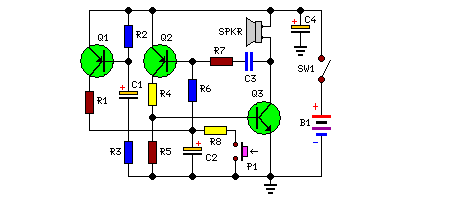# Learning Electronics

Learn to build electronic circuits

# Powerful Security Siren

Simple circuit - No ICs required, 12V Battery operation

This circuit was requested by several correspondents. Its purpose was to obtain more power than the siren circuit already available on this website (One-IC two-tones Siren) and to avoid the use of ICs. A complementary transistor pair (Q2 & Q3) is wired as a high efficiency oscillator, directly driving the loudspeaker. Q1 ensures a full charge of C2 when power is applied to the circuit. Pressing on P1, C2 gradually discharges through R8: the circuit starts oscillating at a low frequency that increases slowly until a high steady tone is reached and kept indefinitely. When P1 is released, the output tone frequency decreases slowly as C2 is charged to the battery positive voltage through R6 and the Base-Emitter junction of Q2. When C2 is fully charged the circuit stops oscillating, reaching a stand-by status.

Circuit Diagram:Large View

Parts:

P1 = SPST Pushbutton Operating Switch
R1 = 1K
R2 = 10K
R3 = 1K
R4 = 220R
R5 = 10K
R6 = 220K
R7 = 22K
R8 = 100K
C1 = 22uF-25V
C2 = 22uF-25V
C3 = 10nF-63V
C4 = 47uF-25V
Q1 = BC557
Q2 = BC557
Q3 = BC337
B1 = 12V Battery
SW1 = SPST Toggle or Slide Main Switch
SPKR = 8 Ohms Loudspeaker

Notes:
• A good sized loudspeaker will ensure a better and powerful output tone.
• As stand-by current drawing is zero, SW1 can be omitted and B1 wired directly to the circuit.
• Maximum current drawing at full output is about 200mA.

Source : www.redcircuits.com